Rs Aggarwal 2017 Solutions for Class 8 Math Chapter 23 Pie Charts are provided here with simple step-by-step explanations. These solutions for Pie Charts are extremely popular among Class 8 students for Math Pie Charts Solutions come handy for quickly completing your homework and preparing for exams. All questions and answers from the Rs Aggarwal 2017 Book of Class 8 Math Chapter 23 are provided here for you for free. You will also love the ad-free experience on Meritnation’s Rs Aggarwal 2017 Solutions. All Rs Aggarwal 2017 Solutions for class Class 8 Math are prepared by experts and are 100% accurate.

#### Question 1:

The monthly income of family is ₹ 28800. The monthly expenditure of the family on various items is given below.

 Item Rent Food Clothing Education Saving Expenditure (in ₹) 8000 10800 5600 3600 800
Represent the above data by a pie chart.

Monthly income of the family = ₹28,800

Calculation of central angles

 Items Expenditure(in ₹) Central angle Rent 8000 100º Food 10800 135º Clothing 5600 70º Education 3600 45º Savings 800 10º

Construction of pie chart
Steps of construction
1. Draw a circle of any convenient radius.
2. Draw a horizontal radius of this circle.
3. Draw sectors whose central angles are 100º, 135º, 70º, 45º and 10º.
4. Shade the sectors so obtained differently and label each one of them.
Thus, the pie chart is obtained as follows.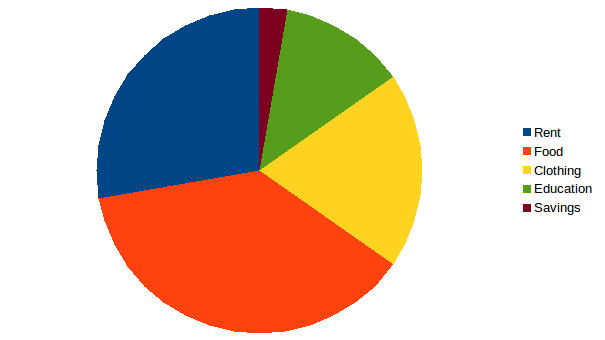#### Question 2:

There are 900 creatures in a zoo as per list given below:

 Beast animals Other land animals Birds Water animals Reptiles 150 400 175 125 50

Represent the above data by a pie chart.

Creatures Number of creatures Central angle
Beast animals 150 $60°$
Other land animals 400 $160°$
Birds 175 $70°$
Water animals 125 $50°$
Reptiles 50 $20°$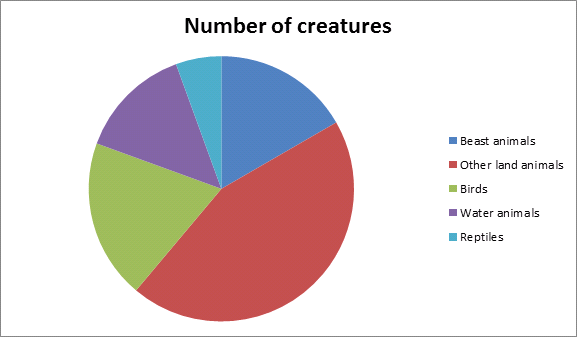#### Question 3:

Various modes of transport used by 1260 students in a given school are given below:

 School bus Private bus Bicycle Rickshaw On foot 350 245 210 175 280

Represent the above data by a pie chart.

Mode of transport Number of students Central angle
School bus 350 $100°$
Private bus 245 $70°$
Bicycle 210 $60°$
Rickshaw 175 $50°$
On foot 280 $80°$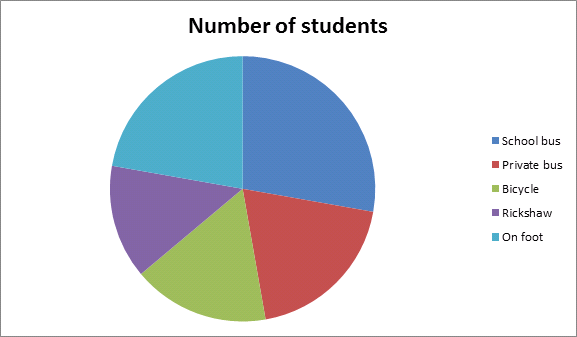#### Question 4:

The data given below shows number of hours spent by a school boy on different activities on a working day.

 Activity School Homework Play Sleep Others Total Number of hours 7 4 2 8 3 24

Represent the above data by a pie chart.

Activity Number of hours Central angle
School 7 $105°$
Home work 4 $60°$
Play 2 $30°$
Sleep 8 $120°$
Others 3 $45°$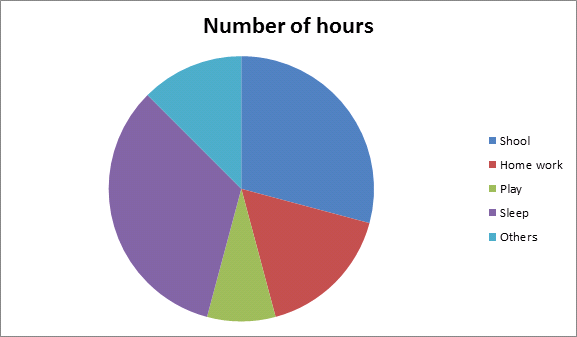#### Question 5:

The data on religion wise division of 1080 workers of a factory are given below:

 Religion Hindu Muslim Sikh Christian Number of workers 450 270 255 105

Draw a pie chart to represent the above data.

Religion Marks obtained Central angle
Hindu 450 $150°$
Muslim 270 $90°$
Sikh 255 $85°$
Christian 105 $35°$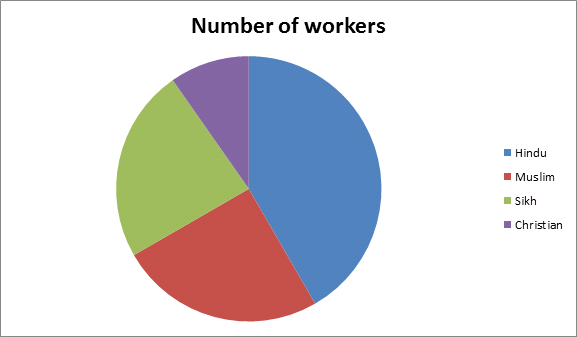#### Question 6:

The marks obtained by Sudhir in an examination are given below:

 Subject English Hindi Mathematics Science Social science Marks obtained 105 75 150 120 90

Represent the above data by a pie chart.

Subject Marks obtained Central angle
English 105 $70°$
Hindi 75 $50°$
Mathematics 150 $100°$
Science 120 $80°$
Social science 90 $60°$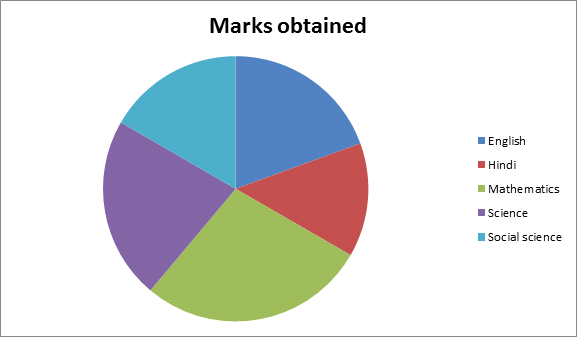#### Question 7:

The following table gives the number of different fruits kept in a hamper.

 Type of fruit Mangoes Apples Oranges Coconuts Pomegranates Number 26 30 21 5 8

Represent the above data by a pie chart.

Types of fruit Number Central angle
Mangoes 26 $104°$
Apples 30 $120°$
Oranges 21 $84°$
Coconuts 5 $20°$
Pomegranates 8 $32°$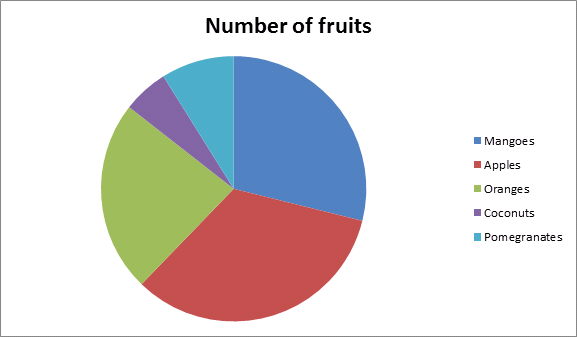#### Question 8:

The following data shows the agricultural production in India during a certain year.

 Foodgrain Rice Wheat Coarse cereals Pulses Production (in millions of tonnes) 57 76 38 19

Draw a pie chart to represent the above data.

Food grain production (in millions of tonnes) Central angle
Rice 57 $108°$
Wheat 76 $144°$
Coarse cereals 38 $72°$
Pulses 19 $36°$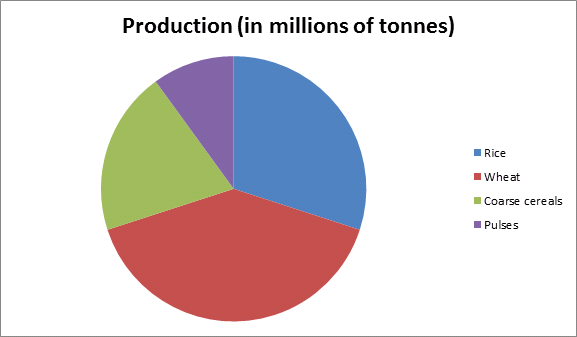#### Question 9:

Given below is the result of an annual examination of a class, showing the percentage of students in each category.

 First division Second division Third division Failed 25% 45% 20% 10%

Represent the above data by a pie chart.

Category Result (in %) Central angle
First division 25 $90°$
Second division 45 $162°$
Third division 20 $72°$
Failed 10 $36°$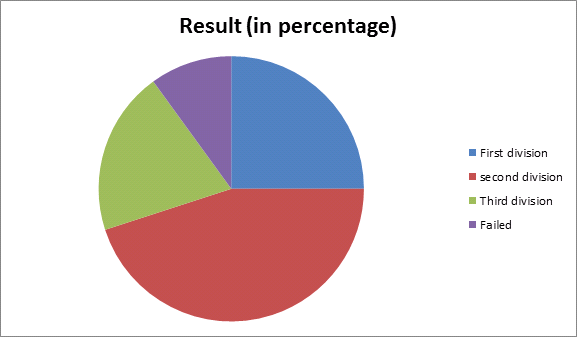#### Question 10:

The following table shows the percentages of buyers of four different brands of bathing soaps.

 Brand A B C D Percentage of buyers 20% 40% 25% 15%

Represent the above data by a pie chart.

Brand Buyers (in %) Central angle
A 20 $72°$
B 40 $144°$
C 25 $90°$
D 15 $54°$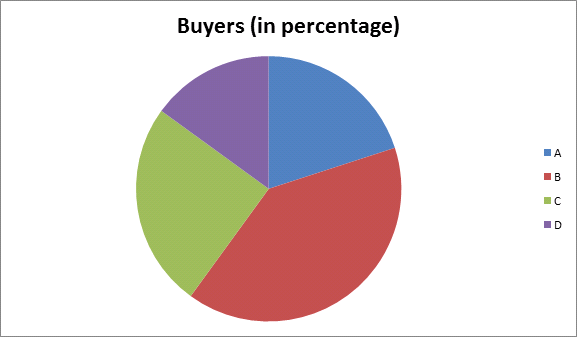#### Question 1:

A man's monthly salary is Rs 2400 and his monthly expenses on travel are Rs 250. The central angle of the sector representing travel expenses in the pie chart would be
(a) 30°
(b) $37\frac{1}{2}°$
(c) 45°
(d) 60°

(b) $37\frac{1}{2}°$

$=\left(\frac{250}{2400}×360\right)°\phantom{\rule{0ex}{0ex}}=37\frac{1}{2}°$

#### Question 2:

If 35% of the people residing in a locality are Sikhs then the central angle of the sector representing the Sikh community in the pie chart would be
(a) 108°
(b) 115°
(c) 126°
(d) 135°

(c) 126°

$=\left(\frac{35}{100}×360\right)°\phantom{\rule{0ex}{0ex}}=126°$

#### Question 3:

If in the pie chart representing the number of students opting for different streams of study out of a total strength of 1650 students, the central angle of the sector representing arts students is 48° then what is the number of students who opted for arts stream?
(a) 220
(b) 240
(c) 275
(d) 320

(a) 220

#### Question 4:

(c) $22\frac{1}{2}%$
(d) $27\frac{1}{2}%$
(c) $22\frac{1}{2}%$